﻿ 一种求解化学非平衡流动的新型解耦算法
«上一篇文章快速检索 高级检索

 力学学报2015, Vol. 47Issue (1): 82-94  DOI: 10.6052/0459-1879-14-0890

### 引用本文 [复制中英文]

[复制中文]
Liu Yu, Liu Jun, Tang Lingyan, Cui Xiaoqiang. A NOVEL UNCOUPLED ALGORITHM FOR SOLVING CHEMICAL NONEQUILIBRIUM FLOWS[J]. Chinese Journal of Ship Research, 2015, 47(1): 82-94. DOI: 10.6052/0459-1879-14-089.
[复制英文]

### 文章历史

2014-04-01 收稿
2014-06-24 录用
2014–11–02 网络版发表

1. 装备学院航天装备系, 北京 101416;
2. 大连理工大学航空航天学院, 大连 116024;
3. 国防科学技术大学理学院, 长沙 410073;
4. 中国人民解放军95972 部队2站, 兰州 735018

Henrick等对经典的5阶WENO格式进行了严格的分析，得到了5阶WENO格式在临界点处具有5阶精度的充分条件，并证明经典的5阶WENO格式在一阶临界点处的收敛精度只有3阶；构造了WENO格式子模板权系数的映射函数，经典的5阶WENO格式的权系数经过映射后更接近于理想权系数，并满足5阶收敛的充分条件，根据这种方法，WENO格式在一阶临界点不会降阶，能够比经典的5阶WENO格式更好地捕捉流场间断，这种改进后的格式被称为WENO5M. Borges等在Henrick 等提出的5阶WENO格式保持5阶收敛的充分条件的基础上，构造了一种高阶光滑指示器，对经典的5阶WENO格式的权系数进行了修正，这种方法称为WENO-Z；证明了WENO-Z格式当$p =1$时，在一阶临界点的收敛阶为4；而当$p=2$时，收敛阶是5，并且论证了WENO-Z格式增加了含有间断子模板的权系数，这是WENO-Z格式能比经典的5阶WENO格式更好地捕捉间断的原因，同时也通过计算说明WENO5M也具有相同的性质. Castro等将WENO-Z格式推广到了所有的奇数阶精度.

1 计算方法 1.1 控制方程

 $\dfrac{\partial {\pmb Q}}{\partial t} + \dfrac{\partial {\pmb F }({\pmb Q})}{\partial x} = {\pmb S}$ (1)

 \begin{align} & Q={{\left( \rho \ \ \rho u\ \ \rho {{e}_{t}}\ \ {{\rho }_{1}}\ \cdots \ {{\rho }_{{{n}_{s}}-1}} \right)}^{\text{T}}} \\ & {{F}^{\prime }}={{\left( \rho u\ \ \rho {{u}^{2}}+p\ \ (\rho {{e}_{t}}+p)u\ \ {{\rho }_{1}}u\ \ \cdots \ \ {{\rho }_{{{n}_{s}}-1}}u \right)}^{\text{T}}} \\ & {{S}^{\prime }}={{\left( \ \ 0\ \ 0\ \ {{\omega }_{1}}\ \ \cdots \ {{\omega }_{{{n}_{s}}-1}} \right)}^{\text{T}}} \\ \end{align}

 $\omega _i = \sum\limits_{j = 1}^{n_r } (\nu"_{ij} - \nu'_{ ij} )\left( k_{fj} \prod\limits_{s = 1}^{n_s }[X_s]^{\nu'_{ sj}}- k_{bj} \prod\limits_{s = 1}^{n_s } [X_s]^{\nu"_{sj}} \right) [M]^{\nu _{jM} }$ (2)

1.2 刘君的化学非平衡流解耦算法

 $e_t = e'_t + \sum\limits_{i = 1}^{n_s } {C_i \left( {\Delta h_{f,298}^0 } \right)_i }$ (3)

 $\dfrac{\partial }{\partial t}(\rho e'_t ) + \dfrac{\partial }{\partial x}\left[{(\rho e'_t + p)u} \right]= - \sum\limits_{i = 1}^{n_s } {\omega _i \left( {\Delta h_{f,298}^0 } \right)_i }$ (4)

 $\gamma = \dfrac{h}{e}$ (5)

 $\gamma' = \dfrac{h'}{e'}$ (6)

 $h' = \sum\limits_{i = 1}^{n_s } {C_i \left( {aT + \dfrac{bT^2}{2} + \dfrac{cT^3}{3} + \dfrac{dT^4}{4}+ \dfrac{eT^5}{5}} \right)} R_i$ (7)
 $e' = h' - RT$ (8)

 $e' = \dfrac{p / \rho }{(\gamma' - 1)}$ (9)
 $h' = \dfrac{\gamma'}{\gamma' - 1}\dfrac{p}{\rho }$ (10)

 $\rho e'_t = \dfrac{p}{\gamma' - 1} + \dfrac{1}{2}\rho u^2$ (11)

 $e_t = \dfrac{p}{(\gamma' - 1)\rho } + \dfrac{u^2}{2} + \sum\limits_{i = 1}^{n_s } {C_i \left( {\Delta h_{f,298}^0 }\right)_i }$ (12)

 $e_t = \dfrac{p}{(\gamma - 1)\rho } + \dfrac{u^2}{2} + \sum\limits_{i = 1}^{\rm nreact} {\beta _i Q_i }$ (13)

 $\dfrac{\partial {\pmb Q}'}{\partial t} + \dfrac{\partial {\pmb F}'({\pmb Q}')}{\partial x} = {\pmb S}'$ (14)
 \begin{align} & Q={{\left( \rho \ \ \rho u\ \ \rho {{{{e}'}}_{t}}\ \ {{\rho }_{1}}\ \cdots {{\rho }_{{{n}_{s}}-1}} \right)}^{\text{T}}} \\ & {{F}^{\prime }}={{\left( \rho u\ \ \rho {{u}^{2}}+p\ \ (\rho {{{{e}'}}_{t}}+p)u\ {{\rho }_{1}}u\ \ \cdots \ \ {{\rho }_{{{n}_{s}}-1}}u \right)}^{\text{T}}} \\ & {{S}^{\prime }}={{\left( 0\ \ 0\ \ -\sum\limits_{i=1}^{{{n}_{s}}-1}{{{\omega }_{i}}{{\left( \Delta h_{f,298}^{0} \right)}_{i}}}\ \ {{\omega }_{1}}\ \ \cdots \ \ {{\omega }_{{{n}_{s}}-1}} \right)}^{\text{T}}} \\ \end{align}
1.3 数值算法

 $\dfrac{\partial {\pmb Q}'}{\partial t} + \dfrac{\partial {\pmb F} ({\pmb Q}')} {\partial x} =0$ (15)

 $\dfrac{\partial {\pmb Q}'}{\partial t} = {\pmb S}'$ (16)

 $Q'^{n + 1} = {\rm L}_c (\Delta t / 2) {\rm L}_f (\Delta t) {\rm L}_c (\Delta t / 2)Q'^n$ (17)

(1) 化学反应部分计算方法

(2) 流动部分计算方法

(3) 新型解耦算法的特点

① 通过引入等效内能和等效比热比，对描述化学非平衡流的控制方程进行了变换；

②对变换后的化学非平衡流控制方程进行算子分裂，得到的流动控制方程组和化学反应动力学方程组分别描述的是多组分冻结流动和等容爆炸过程，算子分裂的物理意义清晰；

③ 由于引入了等效内能和等效比热比，以及关系式 (9)$\sim$(11)，在形式上描述流动部分的控制方程和描述量热完全气体流动的控制方程是一致的，因此可以直接在求解量热完全气体流动的算法上进行很小的改造，就能得到计算冻结流动控制方程的算法；

④ 可以直接采用现有的计算等容爆炸化学反应的程序来计算描述化学反应部分的控制方程，不需做任何修改；

⑤ 流动部分和化学反应部分的求解是相互独立的，因此程序具有很好的模块化特点；

⑥算法实施简单，在已有的量热完全气体流动计算程序和等容爆炸计算程序的基础上，可以很快的将这两种程序结合并改造成化学非平衡流计算程序.

2 算法验证 2.1 Osher-$\!$-Shu问题

 $\rho = 1 + 0.2\sin (5x) ,\ u = 0 ,\ p = 1 ,\ x \geqslant - 4$
 $\rho = 3.857 143 ,\ u = 2.629 369 ,\ p = 31 / 3 ,\ x \leqslant - 4$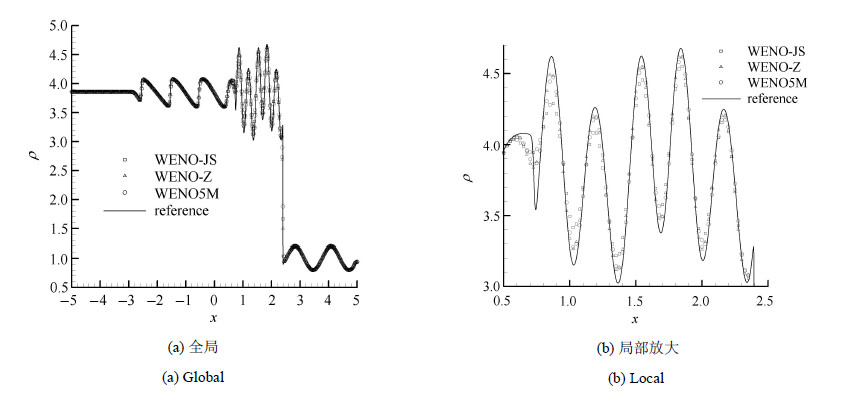图 1 由不同格式计算的密度在$t=1.8$时刻的分布 Fig.1 Calculated density distributions computed by different schemes as $t=1.8$}
2.2 绝热等容爆炸模拟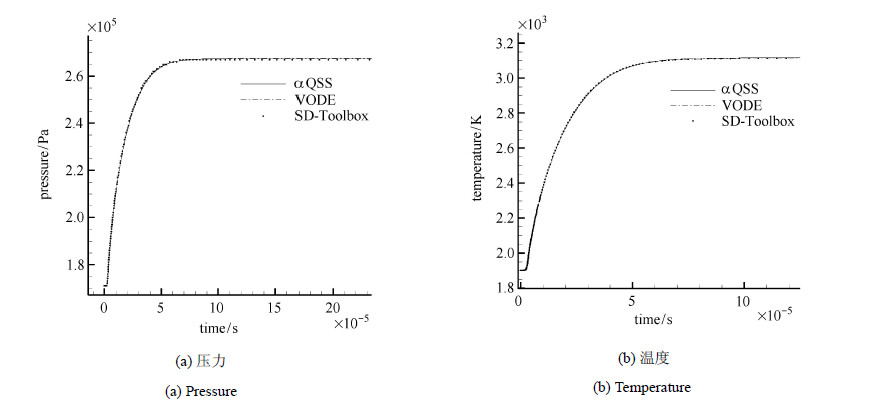图 2 等容爆炸的压力和温度时间历程 Fig.2 The time evolution of pressure and temperature of constant volume explosion
2.3 反射激波诱导爆轰模拟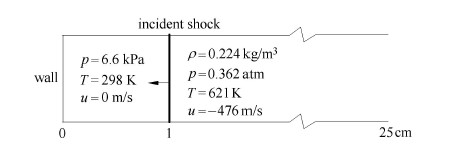图 3 反射激波诱导爆轰波计算域和初始条件示意图 Fig.3 The schematic of computation domain and initial condition for case of detonation induced byreflecting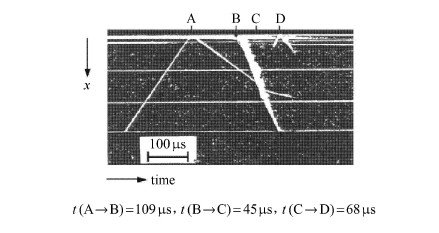图 4 激波管实验时间分辨纹影图 Fig.4 Time-resolved schlieren photograph of shock reflection-reation wave formation process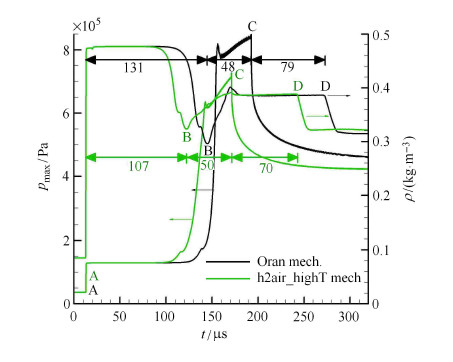图 5 最大压力和壁面密度时变曲线 (Oran机理和h2air\_highT机理的比较) Fig.5 Time evolution of maximum pressure in chamber and density at reflecting wall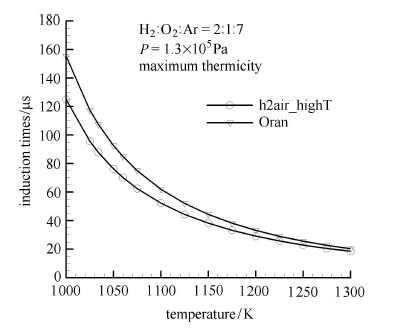图 6 Oran机理和h2air\_highT机理的诱导时间比较 Fig.6 The induction time comparison between Oran and h2air_highT mechanism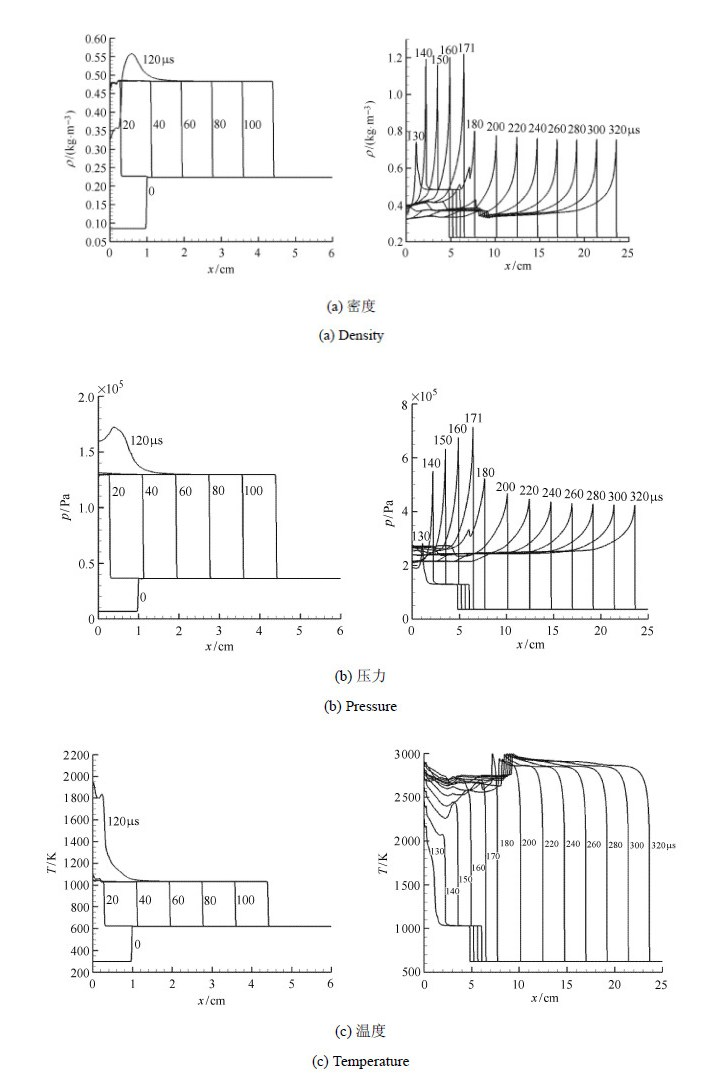图 7 不同时刻密度，压力和温度的空间分布 (h2air_highT机理) Fig.7 Calculated density and pressure andtemperature distributions in chamber at various times (h2air_highT mechanism)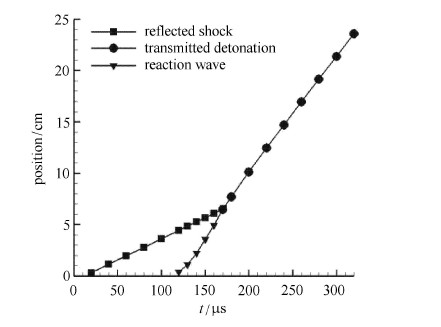图 8 反射激波，反应波和爆轰波阵面位置随时间的变化关系(h2air_highT机理) Fig.8 Calculated position of the reflected shock front, reaction wave, transmitted detonation as afunction of time for ignition behind a reflected shock (h2air_highT mechanism)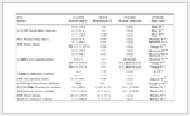表 1 爆轰波速比较 ($\Delta h =0.1$ mm) Table 1 The comparisons of detonation wave speed calculated bydifferent method (the grid spacing is 0.1 mm)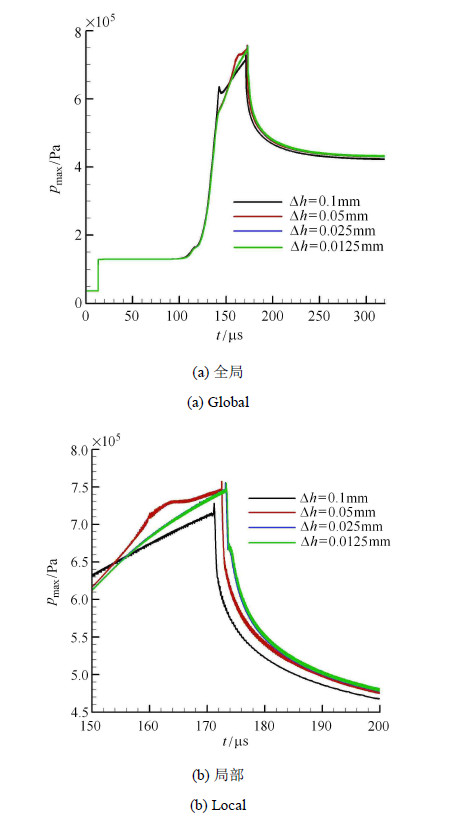图 9 不同网格计算的最大压力时变曲线 (h2air_highT机理) Fig.9 Comparison of time evolution of maximum pressurein chamber with different grid spacing (h2air_highT mechanism)表 2 同网格计算的爆轰波速 (h2air_highT机理) Table 2 The calculated detonation speed via different grid spacing (h2air_highT mechanism
2.4 H2/O2/Ar二维爆轰模拟}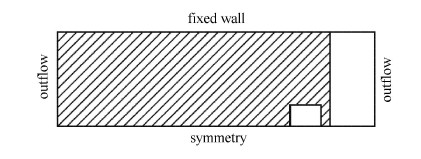图 10 二维爆轰波计算域示意图 Fig.10 The computation domain of 2D detonatio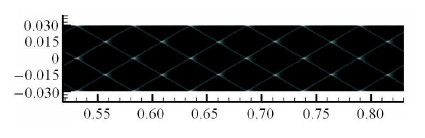图 11 计算的胞格结构(长度单位:m) Fig.11 The calculated detonation cell structure (length unit: m)表 3 胞格尺寸与文献比较 Table 3 The comparisons of cell length and width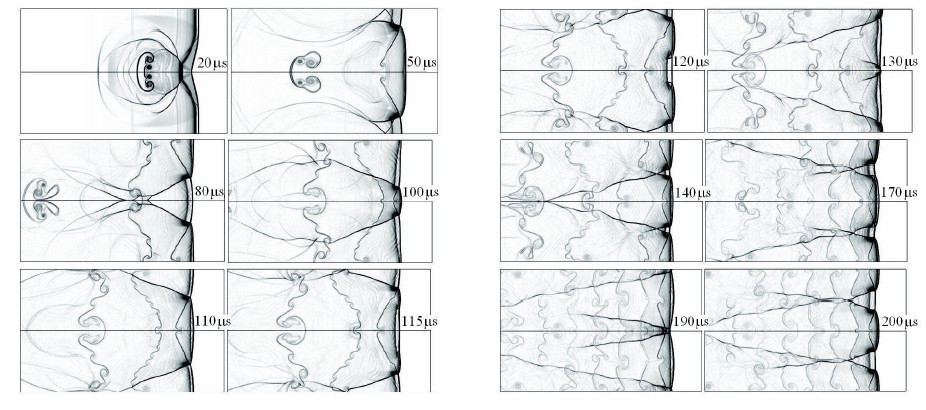图 12 二维爆轰数值纹影图 Fig.12 The numerical schelieren of 2D detonation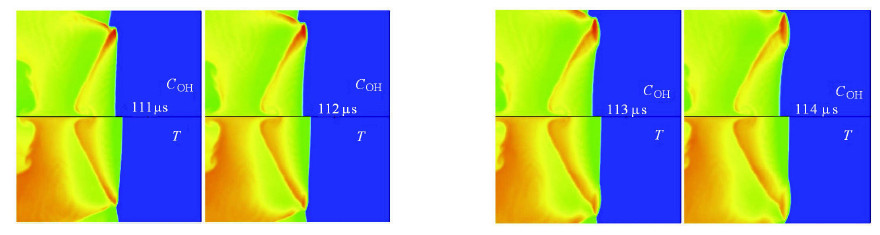图 13 新的三波点形成过程中，OH的质量分数和温度的云图 Fig.13 The contours of mass fraction of OH and temperature in the process of the developelemt of the new triple point
3 结 论

  Choi J-Y, Jeung I-S, Yoon Y. Computational fluid dynamics algorithns for unsteady shock-induced combustion, part 1: validation. AIAA Journal, 2000, 38(7): 1175-1185.  Kirk BS, Stognery RH, Olivery TA, et al. Recent advancements in fully implicit numerical methods for hypersonic reacting flows. AIAA 2013-2559, 2013  Bussing TRA, Murman EM. A finite volume method for the calculation of compressible chemically reaction flows. AIAA-85-0331, 1985  杨顺华, 乐嘉陵, 赵慧勇等. 煤油超然冲压发动机三维大规模并行数值模拟. 计算物理, 2009, 26(4): 534-540 (Yang Shunhua, Le Jialing, Zhao Huiyong, et al. Three-dimensional massively parallel numerical simulation of kerosene-fueled scramjet. Chinese Journal of Computational Physics, 2009, 26(4): 534-540 (in Chinese))  Zhong X. Additive semi-implicit Runge-Kutta methods for computing high-speed nonequilibrium reactive flows. Journal of Computational Physics, 1996, 128(1): 19-31  王昌建, 徐胜利. 直管内胞格爆轰的基元反应数值研究. 爆炸与冲击, 2005, 25(5): 405-416 ( Wang Changjian, Xu Shengli. Numerical study on cellular detonation in a straight tube based on detailed chem ical reaction model. Explosionand Shock Waves, 2005, 25(5): 405-416 (in Chinese))  Mevel R, Davidenko D, Austin JM, et al. Application of a laser induced fluorescence model to the numerical simulation of detonation waves in hydrogene-oxygene-diluent mixtures. International Journal of Hydrogen Energy, 2014, 39(11): 6044-6060  Leveque RJ. Finite Volume Methods for Hypersonic Problems. Cambridge, UK: Cambridge University Press, 2004  Ferrer PJM, Buttay R, Lehnasch G, et al. A detailed verification procedure for compressible reactive multicomponent Navier-Stokes solvers. Computers & Fluids, 2014, 89: 88-110  潘振华, 范春宝, 张旭东等. 连续旋转爆轰三维流场的数值模拟. 兵工学报, 2012, 33(5): 594-599 (Pan Zhenhua, Fan Chunbao, Zhang Xudong, et al. Numerical simulation of three-dimensional flow field of continuous rotating detonation. Acta Armamentarii, 2012, 33(5): 594-599 (in Chinese))  Liu Y, Liu X. Detonation propagation characteristic of H2-O2-N2 mixture in tube and effect of various initial conditions on it. International Journal of Hydrogen Energy, 2013, 38(30): 13471-13483  Taylor BD, Kessler DA, Gamezo VN, et al. Numerical simulations of hydrogen detonations with detailed chemical kinetics. Proceedings of the Combustion Institute, 2013, 34(2): 2009-2016  Uemura Y, Hayashi AK, Asahara M, et al. Transverse wave generation mechanism in rotating detonation. Proceedings of the Combustion Institute, 2013, 34(2): 1981-1989  Tsuboi N, Morii Y, Hayashi AK. Two-dimensional numerical simulation on galloping detonation in a narrow channel. Proceedings of the Combustion Institute, 2013, 34(2): 1999-2007  刘君, 刘瑜, 周松柏. 基于新型解耦算法的激波诱导燃烧过程数值模拟. 力学学报, 2010, 42(3): 572-577 (Liu Jun, Liu Yu, Zhou Songbai. Simulation of shock induced combustion based on a novel uncoupled method. Chinese Journal of Theoretical and Applied Mechanics, 2010, 42(3): 572-578 (in Chinese))  刘君, 张涵信, 高树椿. 一种新型的计算化学非平衡流动的解耦方法. 国防科技大学学报, 2000, 22(5): 19-23 (Liu Jun, Zhang Hanxin, Gao Shuchun. A new uncoupled method for numerical simulation of nonequilibrium flow. Journal of National University of Defense Technology, 2000, 22(5): 19-22 (in Chinese))  刘君. 冲压加速器非平衡流动数值模拟. 弹道学报, 2002, 14(4): 31-35 (Liu Jun. Numerical study on the non-equilibrium flow of ram accelerator in the combustive mixture gas. Journal of Ballistics, 2002, 14(4): 31-35 (in Chinese))  刘君. 非平衡流计算方法及其模拟激波诱导振荡燃烧. 空气动力学学报, 2003, 21(1): 53-58 (Liu Jun. A new nonequilibrium numerical method and simulation of oscillating shock-induced combustion. Acta Aeronautica et Astronautica Sinica, 2003, 21(1): 53-57 (in Chinese))  刘君. 化学动力学模型对H2/Air超燃模拟的影响. 推进技术, 2003, 24(1): 67-70 (Liu Jun. Numerical study on chemical mechanism in supersonic H2/Air mixture gas flow. Journal of Propulsion Technology, 2003, 24(1): 67-70 (in Chinese))  刘世杰. 连续旋转爆震波结构、转播模态及自持机理研究. 长沙: 国防科学技术大学, 2011 (Liu Shijie. Investigations on the Structure, Rotating Mode and Lasting Mechanism of Continuous Rotating Detonation Wave. Changsha: National University of Defense Technology, 2012 (in Chinese))  刘世杰, 孙明波, 林志勇等. 钝头体激波诱导振荡燃烧现象的数值模拟. 力学学报, 2010, 42(4): 597-605 (Liu Shijie, Sun Mingbo, Lin Zhiyong, et al. Numerical research on blunt body shock-induced oscillating combustion. Chinese Journal of Theoretical and Applied Mechanics, 2010, 42(4): 597-605 (in Chinese))  Liu J, Liu Y, Liu R. New uncoupling finite volume method for simulation of non-equilibrium flow and its application to supersonic combustion instability. Transaction of Nanjing University of Aeronautics & Astronautics, 2013, 30(5)  刘瑜, 刘君, 白晓征. 基于新型非结构有限体积解耦算法的激波诱导燃烧数值模拟. 国防科技大学学报, 2011, 33(6): 139-143 (Liu Yu, Liu Jun, Bai Xiaozheng. Numerical simulation of shock-induced combustion with a new uncoupled algorithm in unstructured finite volume method. Journal of National University of Defense Technology, 2011, 33(6): 139-144 (in Chinese))  Harten A, Engquist B, Osher S. Uniformly high order essentially non-oscillatory schemes, III. Journal of Computational Physics, 1987, 71(2): 231-303  Liu XD, Osher S, Chan T. Weighted essentially non-oscillatory schemes. Journal of Computational Physics, 1994, 115(1): 200-212  Jiang GS, Shu CW. Efficient implementation of weighted ENO Schemes. Journal of Computational Physics, 1996, 126(1): 202-228  Balsara DS, Shu CW. Monotonicity preserving weighted essentially non-oscillatory schemes with increasingly high order of accuracy. Journal of Computational Physics, 2000, 160(2): 405-452  Henrick AK, Aslam TD, Powers JM. Mapped weighted essentially non-oscillatory schemes: achieving optimal order near critical points. Journal of Computational Physics, 2005, 207(2): 542-567  Borges R, Carmona M, Costa B, et al. An improved weighted essentially non-oscillatory scheme for hyperbolic conservation laws. Journal of Computational Physics, 2008, 227(6): 3191-3211  Castro M, Costa B, Don WS. High order weighted essentially non-oscillatory WENO-Z schemes for hyperbolic conservation laws. Journal of Computational Physics, 2011, 230(5): 1766-1792  刘瑜. 化学非平衡流动的计算方法研究及其在激波诱导燃烧现象模拟中的应用. [硕士论文]. 长沙: 国防科学技术大学, 2008 (Liu Yu. Numerical study of chemical nonequilibrium flow and its' application in shock-induced combustion. [Master thesis]. National Univerity of Defense Technology, 2008 (in Chinese))  Shepherd JE. SD-Toolbox http://www2.galcit.caltech.edu/EDL/public/ cantera/html/SD_Toolbox/. 2013.  Oran ES, Boris JP. Numerical methods in reacting flows. AIAA-87-0057, 1987  Im K-S, Yu S-T J, Kim C-K. Application of the CESE mehod to detontion with realistic finite-rate chemistry. AIAA-2002-1020, 2002  Wu Y, Ma F, Yang V. Space-time method for detonation problems with finite-rate chemical kinetics. International Journal of Computational Fluid Dynamics, 2004, 18(3): 277-287  Ng HD. The effect of chemical reaction kinetics on the structure of gaseous detonations. [PhD Thesis]. McGill University, 2005  Oran ES, Weber JM, Stefaniw EI, et al. A numerical study of a two-dimensional H2-O2-Ar detonation using a detailed chemical reaction model. Combustion and Flame, 1998, 113(1-2): 147-163  Deiterding R. Parallel adaptive simulation of multi-dimensional detonation structures. [PhD Thesis]. Branderburg University of Technology Cottbus, 2003  Arienti M, Shepherd JE. A numerical study of detonation diffraction. Journal of Fluid Mechanics, 2005, 529: 117-146
A NOVEL UNCOUPLED ALGORITHM FOR SOLVING CHEMICAL NONEQUILIBRIUM FLOWS
Liu Yu, Liu Jun, Tang Lingyan, Cui Xiaoqiang
1. Department of Space Equipment, Academy of Equipment, Beijing 101416, China;
2. School of Aeronautics and Astronautics, Dalian University of Technology, Dalian 116024, China;
3. College of Science, National University of Defense Technology, Changsha 410073, China;
4. Station No.2 of Group 95792, Lanzhou 735018, China
Fund: The project was supported by the Aeronautical Science Foundation of China (20131463009).
Abstract: The WENO5M scheme is modified to simulate the chemical reacting flow based on a novel uncoupled method for chemical nonequilibrium flow. For validating the new implementation, several benchmarks have been tested. The Oran's shock induced detonation experiment has been simulated first. The influences of different chemical reaction mechanisms and grid convergence have been considered and the results compare well with those from experiments. The second test case is the 2d H2/O2/Ar detonation, and the simulated cell structure compares well with the experiment results and other simulated results. Through these validations, we can conclude that the new implementation of WENO5M scheme for the simulation of chemical reacting flow based on the novel decoupled method is satisfied. Because the extension of original WENO5M scheme to calculate the chemical reacting flow based on the novel algorithm is straightforward and only minor modifications are needed, the advantages of this uncoupled algorithm is obvious.
Key words: chemical reacting flow    uncoupled method    high-order scheme